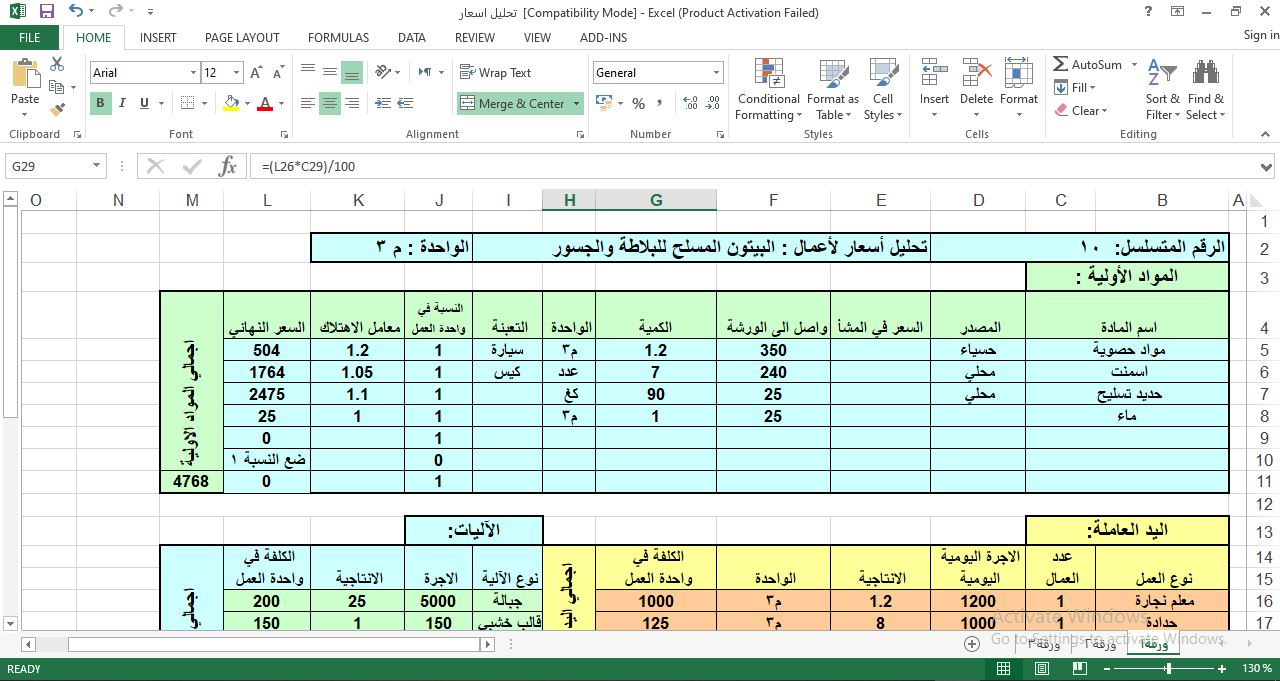House Construction Cost Calculator Excel Sheet

# House Construction Cost Calculator Excel SheetHouse Construction Cost Calculator Excel Sheet

Any construction project consists of direct and indirect costs which forms the total costs and expenses resulting from the use of principal components for implementing construction projects. Different classification of construction project costs and their features are explained below.

#### Types of Construction Project Costs

Before moving into the main classification of project costs, some of the specific costs encountered in construction projects are explained below.

1. Fixed Cost: This is defined as the cost spent once for a particular point of time. The purchase of equipment, machinery etc comes under fixed cost assets.

2. Time-Related Cost: Time-related cost is the cost spend for a particular activity for a given duration. The cost spent on wages, equipment and building rents etc comes under this category.

3. Quantity -Proportional Cost: This type of cost will vary based on the quantities. Materials costs are examples of quantity-proportional costs.